# Projectile Motion JEE MAin(Part - 2) - Physics, Solution by DC Pandey Notes | Study DC Pandey Solutions for JEE Physics - JEE

## JEE: Projectile Motion JEE MAin(Part - 2) - Physics, Solution by DC Pandey Notes | Study DC Pandey Solutions for JEE Physics - JEE

The document Projectile Motion JEE MAin(Part - 2) - Physics, Solution by DC Pandey Notes | Study DC Pandey Solutions for JEE Physics - JEE is a part of the JEE Course DC Pandey Solutions for JEE Physics.
All you need of JEE at this link: JEE

Projectile Motion in Inclined Plane

Ques 15: Find time of flight and range of the projectile along the inclined plane as shown in figure, (g = 10 m/s2)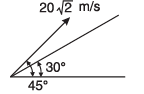Ans: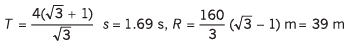Sol: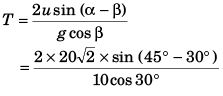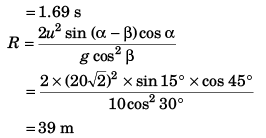Ques 16: Find time of flight and range of the projectile along the inclined plane as shown in figure, (g = 10m/s2)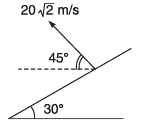Ans: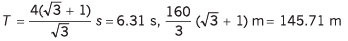Sol: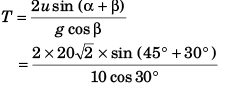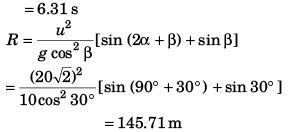Ques 17: Find time of flight and range of the projectile along the inclined plane as shown in figure, (g = 10 m/s2)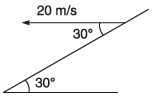Ans: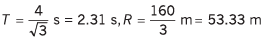Sol: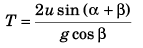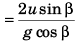(∵ α = 0°)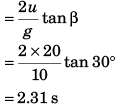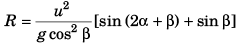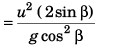[as α = 0°]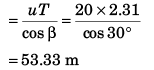Ques 18: A projectile is fired with a velocity u at right angles to the slope, which is inclined at an angle θ with the horizontal. Derive an expression for the distance R to the point of impact.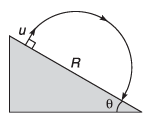Ans: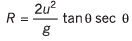Sol: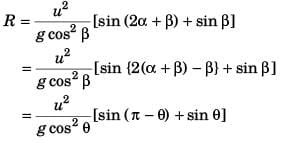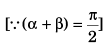or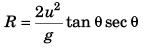Relative Motion in Projectiles
Note:
The problems can also be solved without using the concept of relative motion.
Ques 19: A particle is projected upwards with velocity 20 m/s. Simultaneously another particle is projected with velocity 20√2 m/s at 45°. (g = 10 m/s2)
(a) What is acceleration of first particle relative to the second?
(b) What is initial velocity of first particle relative to the other?
(c) What is distance between two particles after 2 s?
Ans: (a) zero
(b) 20 ms-1 in horizontal direction
(c) 40 m
Sol: (a)  Acceleration of particle 1 w.r.t. that of particle 2
= (- g) - (- g)
= 0
(b) Initial velocity of 1st particle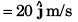Initial velocity of 2nd particle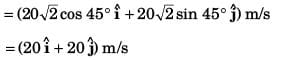∵ Initial velocity of 1st particle w.r.t. that of 2nd particle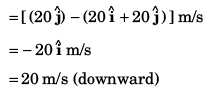(c) Horizontal velocity of 1st particle = 0 m/s
Horizontal velocity of 2nd particle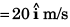∴ Horizontal velocity of 1st particle w.r.t. that of 2nd particle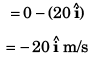Relative displacement of 1st particle w.r.t. 2nd particle at t = 2 s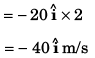∴ Distance between the particles at t = 2 s = 40 m

Ques 20: Passenger of a train just drops a stone from it. The train was moving with constant velocity. What is path of the stone as observed by
(a) the passenger itself,
(b) a man standing on ground?
Ans: (a) A vertical straight line
(b) A parabola
Ans: (a) As observed by passenger Vertical acceleration of stone
= g - 0 = g
Horizontal velocity of stone
= v - v = 0
∴ Path of the stone will be a straight line (downwards).
(b) As observed by man standing on ground
Vertical acceleration of stone = g
Horizontal velocity of stone = v
∴ Path of the stone will be parabolic.

Ques 21: An elevator is going up with an upward acceleration of 1 m/s2. At the instant when its velocity is 2 m/s, a stone is projected upward from its floor with a speed of 2 m/s relative to the elevator, at an elevation of 30°.
(a) Calculate the time taken by the stone to return to the floor.
(b) Sketch the path of the projectile as observed by an observer outdside the elevator.
(c) If the elevator was moving with a downward acceleration equal to g, how would the motion be altered?

Ans: (a) 0.18 s
Sol: (a) geff = g - (- a)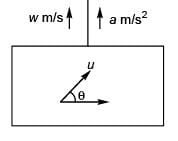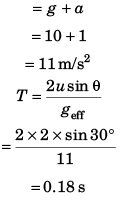(b) Dotted path [(in lift) acceleration upwards]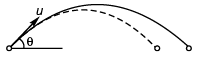Full line path [In lift at rest or moving with constant velocity upwards or downwards].
(c) If lift is moving downward with acceleration g.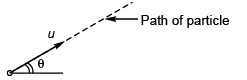geff = g - g = 0

Ques 21: Two particles A and B are projected simultaneously in a vertical plane as shown in figure. They collide at time t in air. Write down two necessary equations for collision to take place.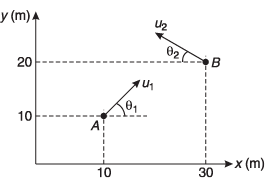Ans: (u1cos θ1 + u2 cos θ2) t = 20 ...(i)
(usinθ- u2 sinθ2) t = 10 ...(ii)
Sol: Horizontal motion:
x1 = u1 cos θ1 and x2 = u2 cos θ
∴ u1 cos θ1 + u2 cos θ2 = 20 …(i)
Vertical motion: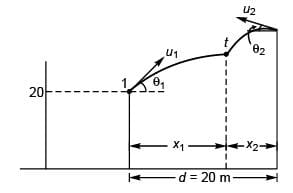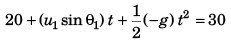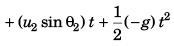or (u1 sin θ1 - u2 sin θ2) t = 10 …(ii)

The document Projectile Motion JEE MAin(Part - 2) - Physics, Solution by DC Pandey Notes | Study DC Pandey Solutions for JEE Physics - JEE is a part of the JEE Course DC Pandey Solutions for JEE Physics.
All you need of JEE at this link: JEEUse Code STAYHOME200 and get INR 200 additional OFF

## DC Pandey Solutions for JEE Physics

210 docs

Track your progress, build streaks, highlight & save important lessons and more!

,

,

,

,

,

,

,

,

,

,

,

,

,

,

,

,

,

,

,

,

,

,

,

,

;